Anzeige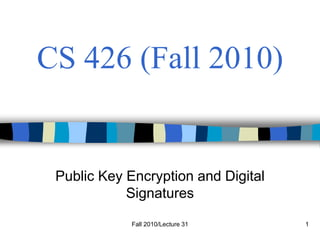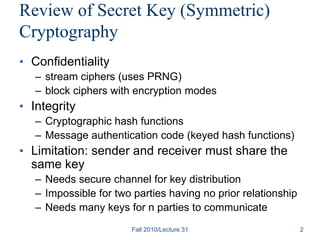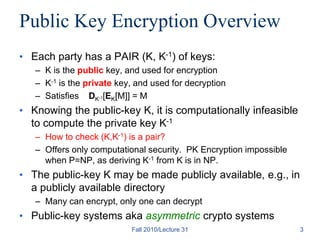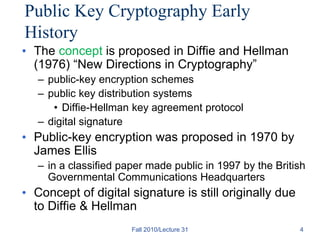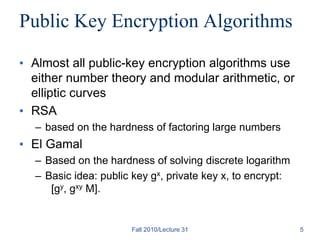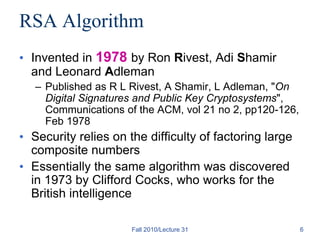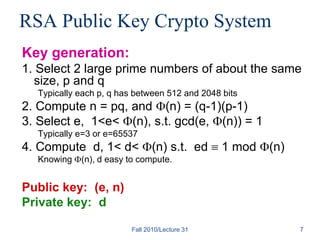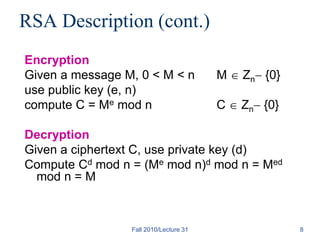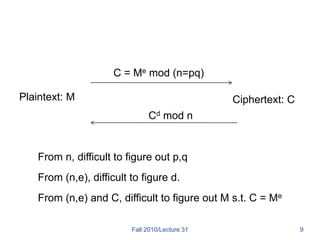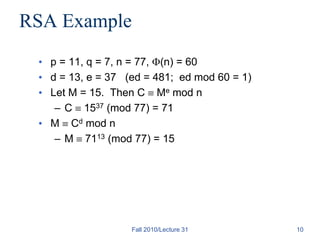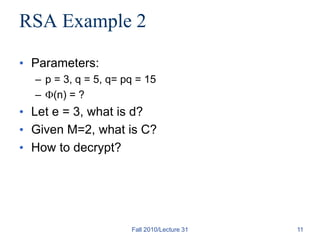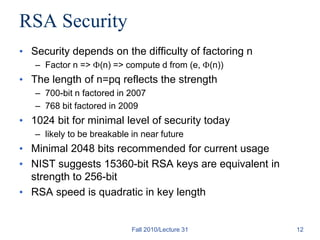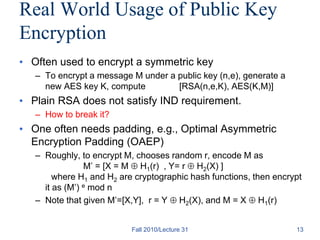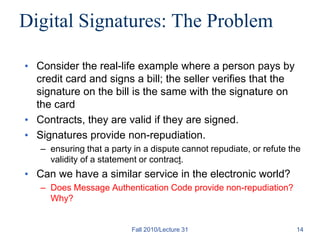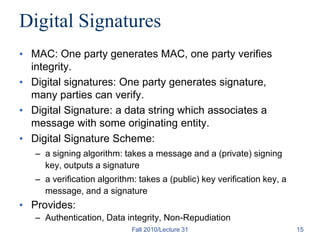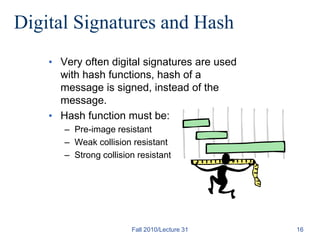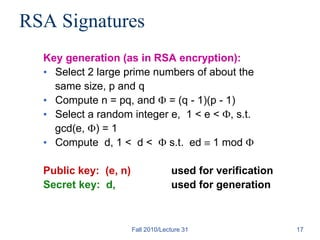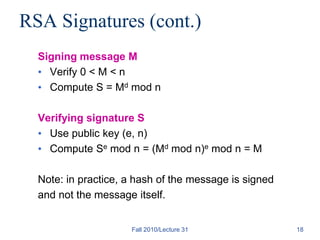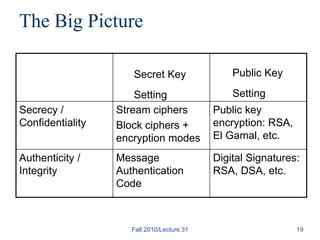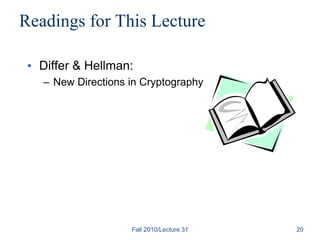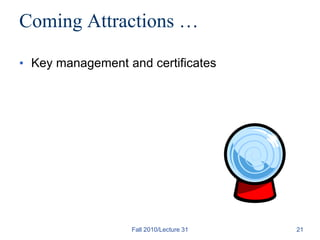1 von 21
Anzeige

### New ppt.ppt

1. Fall 2010/Lecture 31 1 CS 426 (Fall 2010) Public Key Encryption and Digital Signatures
2. Fall 2010/Lecture 31 2 Review of Secret Key (Symmetric) Cryptography • Confidentiality – stream ciphers (uses PRNG) – block ciphers with encryption modes • Integrity – Cryptographic hash functions – Message authentication code (keyed hash functions) • Limitation: sender and receiver must share the same key – Needs secure channel for key distribution – Impossible for two parties having no prior relationship – Needs many keys for n parties to communicate
3. Fall 2010/Lecture 31 3 Public Key Encryption Overview • Each party has a PAIR (K, K-1) of keys: – K is the public key, and used for encryption – K-1 is the private key, and used for decryption – Satisfies DK-1[EK[M]] = M • Knowing the public-key K, it is computationally infeasible to compute the private key K-1 – How to check (K,K-1) is a pair? – Offers only computational security. PK Encryption impossible when P=NP, as deriving K-1 from K is in NP. • The public-key K may be made publicly available, e.g., in a publicly available directory – Many can encrypt, only one can decrypt • Public-key systems aka asymmetric crypto systems
4. Fall 2010/Lecture 31 4 Public Key Cryptography Early History • The concept is proposed in Diffie and Hellman (1976) “New Directions in Cryptography” – public-key encryption schemes – public key distribution systems • Diffie-Hellman key agreement protocol – digital signature • Public-key encryption was proposed in 1970 by James Ellis – in a classified paper made public in 1997 by the British Governmental Communications Headquarters • Concept of digital signature is still originally due to Diffie & Hellman
5. Fall 2010/Lecture 31 5 Public Key Encryption Algorithms • Almost all public-key encryption algorithms use either number theory and modular arithmetic, or elliptic curves • RSA – based on the hardness of factoring large numbers • El Gamal – Based on the hardness of solving discrete logarithm – Basic idea: public key gx, private key x, to encrypt: [gy, gxy M].
6. Fall 2010/Lecture 31 6 RSA Algorithm • Invented in 1978 by Ron Rivest, Adi Shamir and Leonard Adleman – Published as R L Rivest, A Shamir, L Adleman, "On Digital Signatures and Public Key Cryptosystems", Communications of the ACM, vol 21 no 2, pp120-126, Feb 1978 • Security relies on the difficulty of factoring large composite numbers • Essentially the same algorithm was discovered in 1973 by Clifford Cocks, who works for the British intelligence
7. Fall 2010/Lecture 31 7 RSA Public Key Crypto System Key generation: 1. Select 2 large prime numbers of about the same size, p and q Typically each p, q has between 512 and 2048 bits 2. Compute n = pq, and (n) = (q-1)(p-1) 3. Select e, 1<e< (n), s.t. gcd(e, (n)) = 1 Typically e=3 or e=65537 4. Compute d, 1< d< (n) s.t. ed  1 mod (n) Knowing (n), d easy to compute. Public key: (e, n) Private key: d
8. Fall 2010/Lecture 31 8 RSA Description (cont.) Encryption Given a message M, 0 < M < n M  Zn {0} use public key (e, n) compute C = Me mod n C  Zn {0} Decryption Given a ciphertext C, use private key (d) Compute Cd mod n = (Me mod n)d mod n = Med mod n = M
9. Fall 2010/Lecture 31 9 Plaintext: M C = Me mod (n=pq) Ciphertext: C Cd mod n From n, difficult to figure out p,q From (n,e), difficult to figure d. From (n,e) and C, difficult to figure out M s.t. C = Me
10. Fall 2010/Lecture 31 10 RSA Example • p = 11, q = 7, n = 77, (n) = 60 • d = 13, e = 37 (ed = 481; ed mod 60 = 1) • Let M = 15. Then C  Me mod n – C  1537 (mod 77) = 71 • M  Cd mod n – M  7113 (mod 77) = 15
11. RSA Example 2 • Parameters: – p = 3, q = 5, q= pq = 15 – (n) = ? • Let e = 3, what is d? • Given M=2, what is C? • How to decrypt? Fall 2010/Lecture 31 11
12. Fall 2010/Lecture 31 12 RSA Security • Security depends on the difficulty of factoring n – Factor n => (n) => compute d from (e, (n)) • The length of n=pq reflects the strength – 700-bit n factored in 2007 – 768 bit factored in 2009 • 1024 bit for minimal level of security today – likely to be breakable in near future • Minimal 2048 bits recommended for current usage • NIST suggests 15360-bit RSA keys are equivalent in strength to 256-bit • RSA speed is quadratic in key length
13. Real World Usage of Public Key Encryption • Often used to encrypt a symmetric key – To encrypt a message M under a public key (n,e), generate a new AES key K, compute [RSA(n,e,K), AES(K,M)] • Plain RSA does not satisfy IND requirement. – How to break it? • One often needs padding, e.g., Optimal Asymmetric Encryption Padding (OAEP) – Roughly, to encrypt M, chooses random r, encode M as M’ = [X = M  H1(r) , Y= r  H2(X) ] where H1 and H2 are cryptographic hash functions, then encrypt it as (M’) e mod n – Note that given M’=[X,Y], r = Y  H2(X), and M = X  H1(r) Fall 2010/Lecture 31 13
14. Fall 2010/Lecture 31 14 Digital Signatures: The Problem • Consider the real-life example where a person pays by credit card and signs a bill; the seller verifies that the signature on the bill is the same with the signature on the card • Contracts, they are valid if they are signed. • Signatures provide non-repudiation. – ensuring that a party in a dispute cannot repudiate, or refute the validity of a statement or contract. • Can we have a similar service in the electronic world? – Does Message Authentication Code provide non-repudiation? Why?
15. Fall 2010/Lecture 31 15 Digital Signatures • MAC: One party generates MAC, one party verifies integrity. • Digital signatures: One party generates signature, many parties can verify. • Digital Signature: a data string which associates a message with some originating entity. • Digital Signature Scheme: – a signing algorithm: takes a message and a (private) signing key, outputs a signature – a verification algorithm: takes a (public) key verification key, a message, and a signature • Provides: – Authentication, Data integrity, Non-Repudiation
16. Fall 2010/Lecture 31 16 Digital Signatures and Hash • Very often digital signatures are used with hash functions, hash of a message is signed, instead of the message. • Hash function must be: – Pre-image resistant – Weak collision resistant – Strong collision resistant
17. Fall 2010/Lecture 31 17 RSA Signatures Key generation (as in RSA encryption): • Select 2 large prime numbers of about the same size, p and q • Compute n = pq, and  = (q - 1)(p - 1) • Select a random integer e, 1 < e < , s.t. gcd(e, ) = 1 • Compute d, 1 < d <  s.t. ed  1 mod  Public key: (e, n) used for verification Secret key: d, used for generation
18. Fall 2010/Lecture 31 18 RSA Signatures (cont.) Signing message M • Verify 0 < M < n • Compute S = Md mod n Verifying signature S • Use public key (e, n) • Compute Se mod n = (Md mod n)e mod n = M Note: in practice, a hash of the message is signed and not the message itself.
19. Fall 2010/Lecture 31 19 The Big Picture Secrecy / Confidentiality Stream ciphers Block ciphers + encryption modes Public key encryption: RSA, El Gamal, etc. Authenticity / Integrity Message Authentication Code Digital Signatures: RSA, DSA, etc. Secret Key Setting Public Key Setting
20. Fall 2010/Lecture 31 20 Readings for This Lecture • Differ & Hellman: – New Directions in Cryptography
21. Fall 2010/Lecture 31 21 Coming Attractions … • Key management and certificates
Anzeige